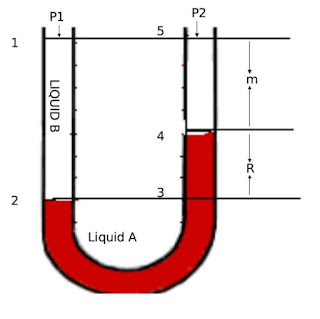Type Here to Get Search Results !
×
Hi ,

We always try to serve you better. Please enter the institute name, you belong to.

×
Hello ,

# Flow of Fluids, Pharmaceutical Engineering, B.Pharmacy As per PCI Syllabi

## Flow of Fluids

### Content: Types of manometers, Reynolds numbers and its significance, Bernoulli's theorem and its applications. Energy losses, Orifice meter, Venturimeter, Pitot tube and Rotameter.

Part 1:- Types of manometers
Part 2:- Reynolds numbers and its significance
Part 3:- Bernoulli's theorem and its applications
Part 4:- Energy losses, Orifice meter, Venturimeter, Pitot tube and Rotameter.

### Manometers:Manometers are the devics used for measuring the pressure differences. Pressure differences based on the principle of balancing the column of liwud by the same or another column of liquids. Three different manometes are available are:
1. Simple Manometes
2. Differential Manometers
3. Inclined Manometers

### Simple Manometers

Simple manometers is a device which measures pressure at a point in a fluid contained pipe or vessel.• Most commonly used manometer
• it consists of a glass U shaped tube filled with a liquid A of density ρA kg /meter cude and above A the arms are filled with liquid B of density ρB.
• The liquid A and B are immiscible and the interface can be seen clearly.
• If two different pressures are applied on the two arms the meniscus on the one liquid will be higher than the other.
• Let pressure at point 1 will be P1 Pascal's and point 5 will be P2 Pascal's
THe pressure at point 2 can be wirtten as

=P1 + (m + R)ρBg
Since  ΔP =  Δ hρg
(m+R) = distance from 3 to 5.
Since the points 2 nad 3 are at same height the pressure.
Pressure at 3P1(m + R)ρBg
Pressure at 4 is less than pressure ar point 3 by RρAg.
Pressure at 5 is still less thatn pressure point 4 by mρBg.

Form all above equations:

P1 + (m + R)ρBg - RρAg - mρBg =  P
ΔP = PP2 = R( ρA - ρB)g

#### Applications:

• Pressure difference can be determined by measuring R.
• Manometer are use in measuring flow of fluid.
• Simple Manometer helps in measuring the consumption of gases in the chemical reactions.
• Manometers are used in conjuction with flow meters for the measurement of flow of fluids. For example, Venturi meter and orifice meter are used for the measurment of pressure head using a manometer. Pitot tube measure the velocity head using manometer.

### Differential Manometers

Differential Manometer is manometer which measure the difference of pressure between any two points in a pipe or vessel containing fluid.
• Diffenrential manometers find occasional application.
• This is suitable for measurement of small pressure differences.
• Sensitive device and useful for measuring even small gas pressure.
Pressure at
Point 1 = P1
Point 2 = P1 + aρBg / gc
Point 3 = P1 + aρBg / gc + bρAg / gc
Point 4 = P1 + aρBg / gc + bρAg / gc
Point 5 = P1 + aρBg / gc + bρAg / gc  - RρCg / gc
Point 6 = P1 + aρBg / gc +  bρAg / gc  - RρCg / gc - dρAg / gc
Point 7 = P1 + aρBg / gc +  bρAg / gc  - RρCg / gc - dρAg / gc - aρBg / gP2

The last equation may simplified to
P1 - P2 = (d - b)ρAg / gc RρCg / gc
but since b - d = R, or fd - b = -R,
ΔP = PP2 = R( ρC - ρA)g / gc
Hence smaller the difference between ρC and ρlarger will be R.
Applications:
• Micromanometers based  on the liquid column principle are available commercially.
• It measure the reading with extreme precision and sensitivity.
• These are free from errors due to capillary and require no calibration, apart from checking the micrometer scale.

### Inclined Tube Manometers

Inclined Tube Manometers or Inclined Manometers is a manometer which measures the minute pressure differences between any two points in a fluid contained in a pipe or vessel.
• To measure small pressure differences need ot magnify Rm, some way.